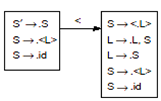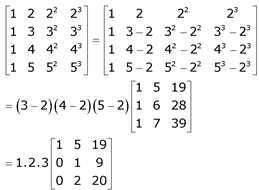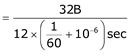# GATE Solved Paper 2017-19 - GATE 2019

 Process P1 P2 P3 P4 Arrival time 0 1 3 4 CPU burst time 3 1 3 Z
These processes are run on a single processor using preemptive Shortest Remaining Time First scheduling algorithm. If the average waiting time of the processes is 1 millisecond, then the value of Z is________.

Note – Numerical Type question

• A

2• B

3• C

1• D

4• Option : A
• Explanation :
Let’s assume Z = 2, then gantt chart will be,  P1 P2 P1 P1 P4 P3 0 1 2 3 4 6 9

Average waiting time,
= {(4-0-3) + (2-1-1) + (9-3-3) + (6-4-2)} / 4
= (1 + 0 + 3 + 0) / 4
= 4 / 4
= 1
So, answer is 2.

• A

4• B

2• C

1• D

0.50• A

3• B

5• C

4• D

2• Option : B
• Explanation :Total number of items in the set GOTO
(I0, 〈 ) is 5.

The absolute value of the product of Eigen values of R is ____________.

Note – Numerical Type question

• A

13• B

15• C

12• D

6• Option : C
• Explanation :
Product of eigenvalues is same as the determinant of a matrix.= 1.2.3.2 = 12

• A

160• B

128• C

256• D

320• Option : A
• Explanation :
Total time to transfer a cache block = 1 + 3 + 8 = 12 cycles
8 W _____________ 12 cycles
8 × 4 bytes ________________ 12 cycles
? B _____________ 1 sec= 160 x 106
Related Quiz.
GATE 2019